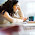## Tuesday, April 28, 2015

### Computing The Fit Of An MDS Solution Using R

If you want to use R2 to evaluate the fit of your MDS solution using the statistical programming language R, then read on.  Some example code is given below. An R script containing the code is available here: http://www.bcp.psych.ualberta.ca/~mike/BlogStuff/RScripts/MDS-Fit-Eg.R

Recently I have been doing a lot of analysis of artificial neural networks that have been trained on a variety of musical tasks.  At many times this analysis has involved computing the distances between different processing units, where these distances are based on connection weights.  Then I perform multidimensional scaling (MDS) on the computed distances .  For the most part I have been performing my MDS analyses in R, using the cmdscale command.

When one performs MDS one typically is doing so to reduce the dimensionality of the distances in order to make the data more understandable.  In MDS you decide how many dimensions you want in order to fit the data.  One important aspect of this is deciding how many dimensions are needed to give a proper fit to your distance data.

In R cmdscale will generate, if asked, a goodness of fit measure that is based on the eigenvalues of the MDS solution.  This goodness of fit measure doesn’t make any sense to me.  Being old school, I would basically like to measure goodness of fit by taking the original distances that I analyze and compare them to the new distances that can be determined from my MDS solution.  In the old days, this was done by taking the two matrices of distances, stringing them out into two columns, and computing the correlation between the two columns.  If you square this correlation, you get R2 which measures the proportion of variance in your original data that is accounted for by your MDS solution.  You can also compute an F statistic from this information .  F = (R2/1)/((1- R2)/(N – 2)) where N is the number of rows in your strung out distance matrix.  The F can then be evaluated at 1, N – 2 degrees of freedom.  You can also use this approach to determine if taking out another dimension accounts for a significant increase in variance accounted for – but see Pedhazur for the details of this approach (in the context of multiple regression).

Unfortunately R does not deliver this old school measure of fit, even though a search of the internet for how to do so reveals that many people seek it.  These poor people, probably old school like myself, are simply told to use the eigenvalue-based goodness of fit that cmdscale will deliver if prompted.

I recently rejected this advice, and wrote some R code to compute the “old school” goodness of fit measure.  I provide this code below; I hope that someone will find it of use!

#Example of calculating goodness of fit
#for MDS via cmdscale()
#Written by Michael R.W. Dawson, spring of 2015

#Basic idea: Do MDS on distance data with cmdscale.  Use the coordinates
#from this solution to recreate the distances.  String the original
#distances and the new distances matrices out into two columns.
#Calculate the correlation between these two columns.  Square this
#correlation to get proportion of variance accounted for.
#Compute degrees of freedom = df = n -2 where n = length of a strung out matrix
#Compute F = (rsquared/1)/((1 - rsquared)/df)
#Evaluate p value for F at 1,df degrees of freedom

#This example uses the eurodist dataset, already a set of distances
#The distances between 21 European cities, standard dataset in R

eurodist #display the starting distance data

# Perform MDS on the data using cmdscale; k determines the number of dimensions
EuroMDS <- cmdscale(eurodist, eig=TRUE, k=2)

# ---- Code below calculates goodness of fit ----

# Copy coordinates from MDS solution into a new matrix
NewEuroCoords = EuroMDS\$points #take coords from cmdscale solution

#Compute a new set of distances from the MDS coordinates delivered by cmdscale
NewEuroDists <- dist(NewEuroCoords, diag=TRUE, upper=TRUE)

#String the two distance matrices out into two columns
#and calculate the correlation between these two columns
r <- cor(c(eurodist), c(NewEuroDists))

#compute and display r squared from this correlation,
#this is variance accounted for
rsquared <- r * r  #compute
rsquared   #display

#compute F test on this correlation

#degrees of freedom for numerator = 1
#compute degrees of freedom for denominator = N - 2 and display
freedom = NROW(c(NewEuroDists)) - 2 #compute
freedom #display df for denominator

#compute and display F
Fvalue <- rsquared /((1 - rsquared)/freedom) #compute
Fvalue #display

#use pf to determine the p-value of this F at df = 1, freedom
pf(Fvalue, 1, freedom, lower.tail=FALSE)

#End result: goodness of fit measured as ability to
#reconstruct original distances, along with
#a significance test of this ability
#Change k to see fit of a different sized
#solution

References

Kruskal, J. B., & Wish, M. (1978). Multidimensional Scaling. Beverly Hills, CA: Sage Publications.

1.thank you, this is useful! is there a similar version to get the r squared from a cluster analysis?

2.Many thanks, simple but useful. Don't know why it isn't in the base code!

3.Thank you! The "GOF" output from cmdscale was driving me insane. Your approach makes so much sense! I agree with aulay, it should be already in the base code!

4.well explained .Keep updating Artificial intelligence Online Trining